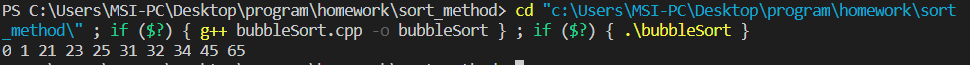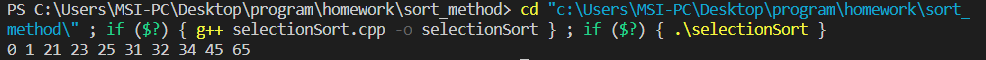# 1. 冒泡排序

## 程序实现

#include <iostream>
using namespace std;

template <typename T>
void bubble_sort(T arr[], int size)
{
T temp;
for (int i = 0; i < size - 1; i++)
for (int j = 0; j < size - i - 1; j++)
{
if (arr[j] > arr[j + 1])
{
temp = arr[j];
arr[j] = arr[j + 1];
arr[j + 1] = temp;
}
}
}

int main()
{
int testdata = {23, 1, 34, 65, 0, 21, 32, 45, 31, 25};
bubble_sort(testdata, sizeof(testdata) / sizeof(int));
for (int i = 0; i < 10; i++)
cout << testdata[i] << ' ';
cout << endl;
return 0;
}

## 运行结果# 2. 选择排序

## 实现流程

• 线性搜索数列并找到最小值
• 将最小值替换为列中左端的数字并进行排序（如果最小值已经在左端，则不进行任何操作）
• 重复12，直到所有数字都被排序

## 程序实现

#include <iostream>
using namespace std;

template <typename T>
void selection_sort(T arr[], int size)
{
T temp;
for (int i = 0; i < size - 1; i++) // 从最左端开始选择，每次选择最左端为最小值
{
int min = arr[i], min_index = i;
for (int j = i + 1; j < size; j++)
{
if (min > arr[j])
{
min = arr[j];
min_index = j;
}
}
if (min == arr[i])
continue;
else
{
temp = arr[i];
arr[i] = arr[min_index];
arr[min_index] = temp;
}
}
}

int main()
{
int testdata = {23, 1, 34, 65, 0, 21, 32, 45, 31, 25};
selection_sort(testdata, sizeof(testdata) / sizeof(int));
for (int i = 0; i < 10; i++)
cout << testdata[i] << ' ';
cout << endl;
return 0;
}

## 运行结果# 5. 归并排序

## 算法介绍

Step 1：将n个元素分成两个含n/2元素的子序列

Step 2：用MS将两个子序列递归排序（最后可以将整个原序列分解成n个子序列）

Step 3：合并两个已排序好的序列

## 程序实现

#include <iostream>
using namespace std;

void Merge(int a[], int s, int m, int e, int tmp[])
{
//将数组a的局部a[s, m]和a[m+1, e]合并到tmp，并保证tmp有序，然后拷回a[s, m]
//操作复杂度O(e-m+1)，即O(n)
int pb = 0;
int p1 = s, p2 = m + 1;
while (p1 <= m && p2 <= e)
{
if (a[p1] < a[p2])
tmp[pb++] = a[p1++];
else
tmp[pb++] = a[p2++];
}
while (p1 <= m)
tmp[pb++] = a[p1++];
while (p2 <= e)
tmp[pb++] = a[p2++];
for (int i = s; i <= e; i++)
a[i] = tmp[i - s];
}

void MergeSort(int a[], int s, int e, int tmp[])
{
if (s < e) //否则不需要做任何事情
{
int m = (s + e) / 2;
MergeSort(a, s, m, tmp);
MergeSort(a, m + 1, e, tmp);
Merge(a, s, m, e, tmp);
}
}

int main()
{
int a[] = {13, 27, 19, 2, 8, 12, 2, 8, 30, 89, 14, 2342, 192, 273, 2, 4, 1, 27};
int b;
int size = sizeof(a) / sizeof(int);

MergeSort(a, 0, size - 1, b);

for (int i = 0; i < size; i++)
cout << a[i] << ' ';
cout << endl;
return 0;
}

## 运行结果# 6. 快速排序

## 程序实现

#include <iostream>
using namespace std;

void swap(int &a, int &b)
{
int tmp = a;
a = b;
b = tmp;
}

void QuickSort(int a[], int s, int e)
{
if (s >= e)
return;
int k = a[s];
int i = s, j = e;
while (i != j)
{
while (i < j && a[j] >= k)
j--;
swap(a[i], a[j]);
while (i < j && a[i] <= k)
++i;
swap(a[i], a[j]);
}
QuickSort(a, s, i - 1);
QuickSort(a, i + 1, e);
}

int main()
{
int a[] = {12, 32, 52, 23, 24, 13, 75, 234, 312, 230, 42};
int size = sizeof(a) / sizeof(int);
QuickSort(a, 0, size - 1);
for (int i = 0; i < size; i++)
cout << a[i] << ' ';
cout << endl;
return 0;
}

## 运行结果Last modification：March 22nd, 2020 at 07:06 pm